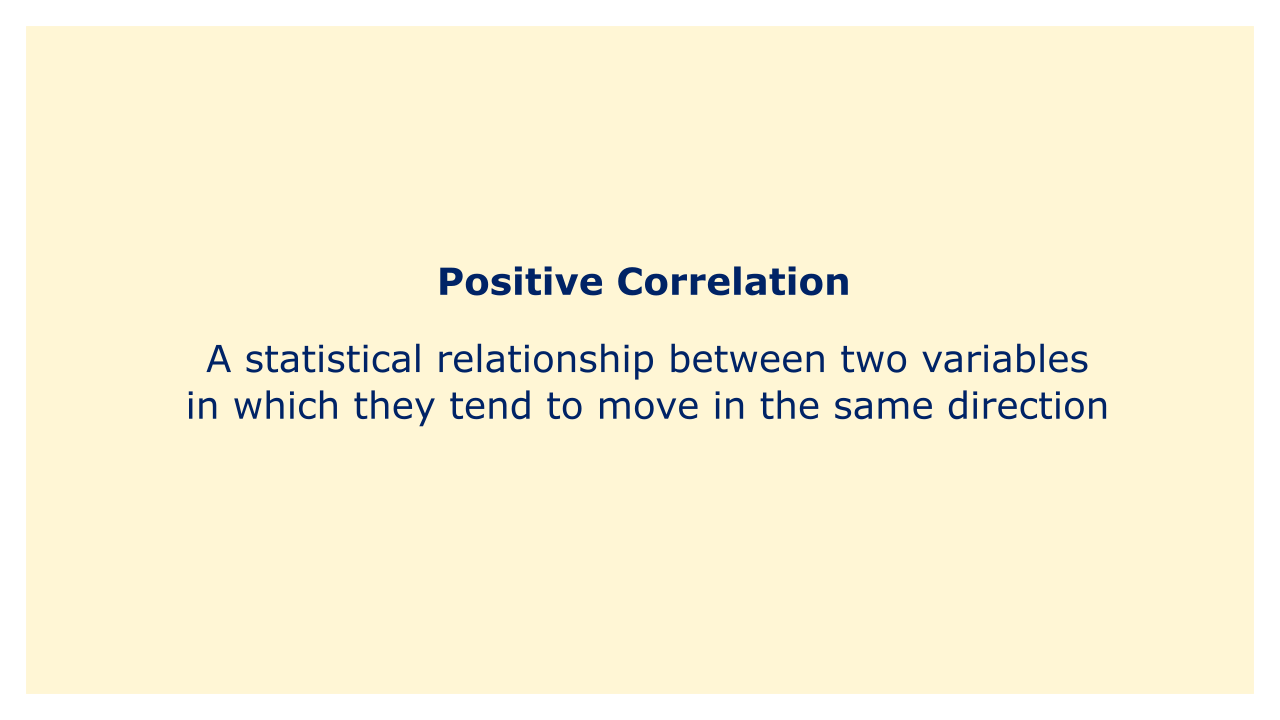# Positive CorrelationImage: Moneybestpal.com

### In statistics, a positive correlation occurs when two variables tend to move in the same direction. This indicates that the tendency is for both variables to rise while one rises. Similar to how one variable tends to drop as another one increases, so does the other.

A positive correlation is frequently used in financial analysis to gauge how closely two financial assets, such as stocks, commodities, or currencies, are related to one another. A correlation coefficient, whose values range from -1 to +1, is used to quantify the strength of the association between two assets. A strong positive correlation between the two assets is indicated by a positive correlation coefficient that is closer to +1, which means that most of the time, the two assets tend to move in the same direction. A weak positive correlation is indicated by a positive correlation coefficient that is closer to 0, which means that the two assets typically move independently of one another most of the time.

By locating financial assets with low positive correlation, or assets that move independently of one another, one can use positive correlation to create a diversified portfolio. This can boost the portfolio's potential return while lowering overall risk. As a result of their propensity to move consistently in the same direction and the potential for increased risk, financial assets with a high degree of positive correlation may be less desirable for inclusion in a diversified portfolio.